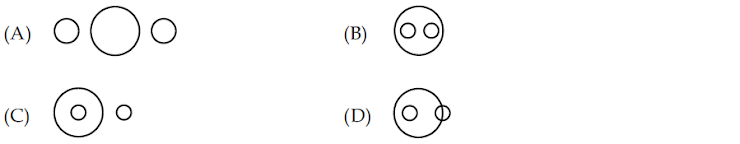UGC-NET/SET-PAPER-I(2008-JUNE) - MODEL PAPER-77
ONLINE TEST NET/SET PAPER-1
Email address *
21. A statistical measure based upon the entire population is called parameter while measure based upon a sample is known as:
2 points
22. The importance of the correlation co-efficient lies in the fact that:
2 points
23. The F-test:
2 points
24. What will be the next letter in the following series: DCXW, FGVU, HGTS, …………
2 points
25. The following question is based on the diagram given below. If the two small circles represent formal class-room education and distance education and the big circle stands for university system of education, which figure represents the university systems.
2 points26. The statement, ‘To be non-violent is good’ is a
2 points
27. Assertion (A) : Man is a rational being. Reason (R) : Man is a social being.
2 points
28. Value Judgements are:
2 points
29. Deductive reasoning proceeds from
2 points
30. AGARTALA is written in code as 14168171, the code for AGRA is:
2 points